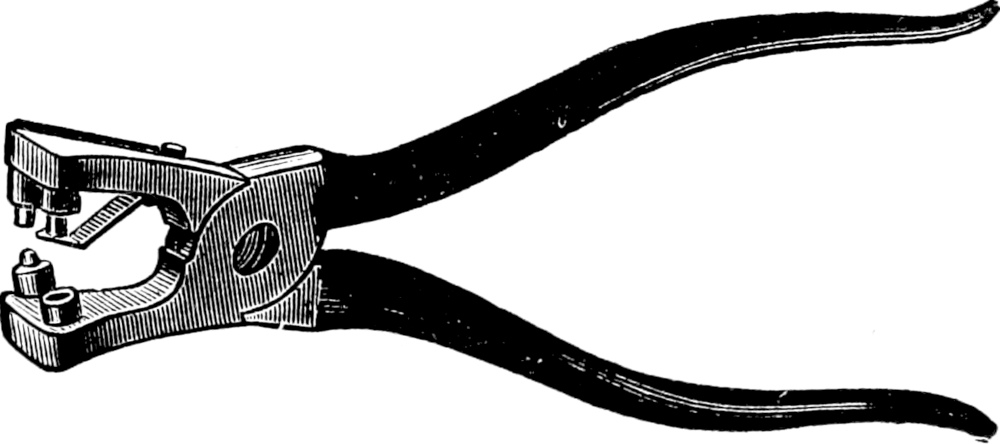# Python Type Hints - How to Use @overloadSometimes the types of several variables are related, such as “if x is type A, y is type B, else y is type C”. Basic type hints cannot describe such relationships, making type checking cumbersome or inaccurate. We can instead use `@typing.overload` to represent type relationships properly.

Take this function:

```from __future__ import annotations

from collections.abc import Sequence

def double(input_: int | Sequence[int]) -> int | list[int]:
if isinstance(input_, Sequence):
return [i * 2 for i in input_]
return input_ * 2
```

The variables have these type relationships:

• If `input_` is an `int`, the return value is an `int`.
• If `input_` is a `Sequence[int]`, the return value is also a `list[int]`.

Only these combinations are possible. It’s not possible for `input_` to be an `int` and the return value to be a `list[int]`, or the opposite. But the current type hints do not capture this relationship.

```x = double(12)
reveal_type(x)
```

Mypy outputs:

```\$ mypy example.py
example.py:11: note: Revealed type is 'Union[builtins.int, builtins.list[builtins.int]]'
```

The input was an `int`, but Mypy has revealed it sees the type of `x` as `int | list[int]` (in the old long-form spelling). Any attempt to use `int`-only operations with `x`, such as division, will fail a type check.

To fix such errors we would be forced to use type narrowing, such as:

```x = double(12)
assert isinstance(x, int)
```

This is quite bothersome to do at every call site.

We can avoid this pain by rewriting the hints for `double` with `@typing.overload` to represent the type relationships:

```from __future__ import annotations

from collections.abc import Sequence
from typing import overload

def double(input_: int) -> int:
...

def double(input_: Sequence[int]) -> list[int]:
...

def double(input_: int | Sequence[int]) -> int | list[int]:
if isinstance(input_, Sequence):
return [i * 2 for i in input_]
return input_ * 2
```

This looks a bit weird at first glance—we are defining `double` three times! Let’s take it apart.

The first two `@overload` definitions exist only for their type hints. Each definition represents an allowed combination of types. These definitions never run, so their bodies could contain anything, but it’s idiomatic to use Python’s `...` (ellipsis) literal.

The third definition is the actual implementation. In this case, we need to provide type hints that union all the possible types for each variable. Without such hints, Mypy will skip type checking the function body.

When Mypy checks the file, it collects the `@overload` definitions as type hints. It then uses the first non-`@overload` definition as the implementation. All `@overload` definitions must come before the implementation, and multiple implementations are not allowed.

When Python imports the file, the `@overload` definitions create temporary `double` functions, but each is overridden by the next definition. After importing, only the implementation exists. As a protection against accidentally missing implementations, attempting to call an `@overload` definition will raise a `NotImplementedError`.

With our type relationship described, let’s check return types for both input types:

```x = double(12)
reveal_type(x)

y = double([1, 2])
reveal_type(y)
```

Mypy says:

```\$ mypy example.py
example.py:23: note: Revealed type is 'builtins.int'
example.py:26: note: Revealed type is 'builtins.list[builtins.int]'
```

Great! The return types align with the input types, as we wanted. `double()` call sites can now be type checked accurately, without any extra narrowing.

`@overload` can represent arbitrarily complex scenarios. For a couple more examples, see the function overloading section of the Mypy docs.

## Fin

May type hints never overload you,